# Operating Principles Of DC Machines Notes | Study Electrical Machines - Electrical Engineering (EE)

## Electrical Engineering (EE): Operating Principles Of DC Machines Notes | Study Electrical Machines - Electrical Engineering (EE)

The document Operating Principles Of DC Machines Notes | Study Electrical Machines - Electrical Engineering (EE) is a part of the Electrical Engineering (EE) Course Electrical Machines.
All you need of Electrical Engineering (EE) at this link: Electrical Engineering (EE)

Operating Principles of DC Machines
(Refer Slide Time: 00:29)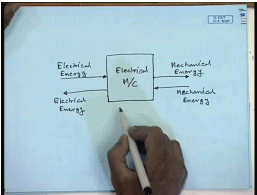Electrical machines particularly rotating electrical machines are basically electro mechanical that is if you have the electrical machine, it can take electrical energy as input, and give out mechanical energy as output , or we can reverse the direction of energy flow that is mechanical energy can be input, and electrical energy can be output, when a electrical machine accepts electrical energy, and gives out mechanical energy we say, it is a motor. When it accepts mechanical energy and gives out electrical energy, it is called a generator. However, it is the same machine the same physical object, which we call the electrical machine performs both these operations. Therefore, every electrical machine can be either a motor or a generator depending on, which is the input, and which is the output.

Now, invariably almost all electrical machines are of rotating type I said almost, because some are not the reason is very simple 1 of compactness in order to either accept or give out mechanical energy. there has to be some motion of some physical object of the machine against an opposing torque. Now, if you want to get continuous mechanical energy output. the motion also has to be continuous and it is convenient, to have a rotating motion which can be continuous, but still be kept within a finite volume that is, why almost all electrical machines are rotating type, now let us see how we can induce a rotation in an electrical machine, the principle is by the principle of magnetic attraction or repulsion let us say this is the inside of a electrical machine.

(Refer Slide Time: 03:29)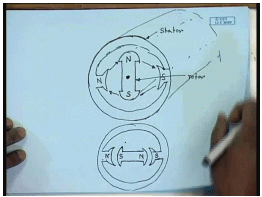So, now at the center of it I have kept a magnet with this north pole, and this as the south pole this is not attached to the other part of the machine, and it is free to rotate therefore, this body is called the rotar and this body, which remain stationary is called the stator every electrical machine will have these 2 comp1 nts.

However, their placement may not exactly be like this there are some construction, where the rotar is outside, while the stator is inside these are called inside out construction. However, normally in more often than not the case will be, where the rotar will be inside the stator. Now, let us see how we can make this rotar rotate here, I would like to mention that we are taking a cross sectional view of a electrical machine. Actually, it is a cylinder kind of a construction, and this rotar also run the whole length of the machine body and normally, hence supported by 2 bearings at the 2 ends. Let, us see, how we can make this run suppose. I somehow create another north pole on the stator on this side, and a south pole on this side joined.

Of course, there will be a force that will be repulsive force on this north pole, there will be a repulsive force on this south pole. There will be attractive force in this direction, and there will be another attractive force in this direction. As, a result this rotar will try to align itself with this north pole and this south pole, and in the aligned position it will look like this. In fact, the rotar will not stop here, because it was moving it will somewhat over soak this position. However, if the stator north and south poles remains this pole maintains this polarity it will be brought back, because there will be opposing restraining force it will be brought back in this position. However, now let us consider a different situation just, when it is aligning let us say I changed the position of the stator poles.

(Refer Slide Time: 07:49)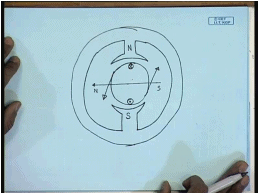Now, I make this south this north, this is south, this was north, what will happen? Now, there will be a repulsive force, which will force this rotar in this direction ,and there will be another repulsive force, which will force this rotar in this direction. So, this magnet will continue to rotate.

Now, there is 1 basic problem to this approach first of all, how do? We shift this polarity of these 2 magnets this can be done without involving physical magnets, if these are electro magnets. We can change this by changing the direction of the current, and that is exactly what is done? In, an electrical machine there is another problem is that, how do we get a magnet on the rotar there are 2 ways of doing. It, 1 is we can use a permanent magnet or we can use another electro magnet in the rotar. In fact, the arrangement can be reversed that is the permanent magnet or the electro magnet can be on permanent magnet may be on the stator, and the electro magnet may be on the rotar this is what is done? In case, of a d c machine which at? Which we will have a slightly more detailed look.

So, let us see the construction of a the cross section of a d c machine. As, I mentioned earlier there are 2 fixed poles for the simplicity. we all assuming only 2 can be more, which are either permanent magnet or electro magnet. Let, us say this is north pole and this is south pole. Inside, we have a cylinder carrying a electro magnet. Let, us say for simplicity made up of only 1 coil. Now, if the current polarity is in this direction that is current is entering through this and coming out through this terminal. Then, it will produce a north pole. In, this the it will produce flux lines in this direction that is this will be an equivalent north pole this will be an equivalent south pole. So, when this is this happens, then there will be a repulsive force acting on this rotation this rotar body in this direction.

(Refer Slide Time: 12:38)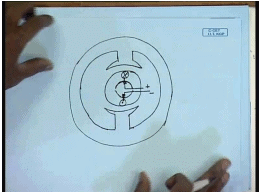And there will be a repulsive force in this direction due to, which this rotar will try to rotate, and at 1 position it will come to the aligned position this is north pole, this is south pole the aligned position it will, become somewhat like this this is the north pole this is the south pole by the rotar electro magnet.

Of course, it will not stop here as before, due to mechanical inertia this will try to over shoot this south pole. Now, this is where we need to change things if, we want to continue if you want to make this rotational continuous. We will need to change either the polarity of this or the polarity of this in a d c machine. It is the polarity of the rotating conductor that is changed that is we make, now this a at this point, we make this a dot, and this a cross. So, that the repulsive and the attractive forces continue in the same direction the device by, which this change of polarity is effected is called a commutator.

(Refer Slide Time: 14:49)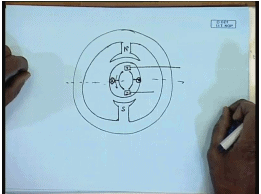So, let us see how a commutator will be placed ((no audio 14:41 to 15:47)). So, these are the conductors, commutator obviously, must be in the same body as in the rotar, because it will make permanent contact with the conductors. Let, us say this is 1commutator segment, this is another commutator segment this conductor connects to this this 1 connects to this. So, this commutator is basically a kind of a ring conducting ring split into 2 parts, in this case and connected to the 2 sides of the coil. However, now I how do I make external connection, how do I make external connection to this commutator that is done through what is called brushes? Which can slide on this commutator ring where should, where should be the position of the brush the position of the brush should be such that, in the aligned position it changes the polarity of the current flowing through them through the coil.

Therefore, here for half the cycle they should connect to 1 commutator for half the cycle should connect to this conductor the same brush should connect to the next other commutator for the rest half. Therefore, we have to connect the brushes on this commutator. So, that when it is in the aligned position it should change, if the brushes are here, which is supplied with a direct voltage. In this figure, we have shown that the cross is connected to the positive terminal the dot is connected to the negative terminal. In this instant, then the this commutator must be connecting to the negative terminal, and this to the positive terminal.

So, this will be the the deduction of rotation is this this should be the negative brush, and this will be the positive brush. So, when it further rotates the cross connector the cross conductor will get connected to the negative brush. Whereas, the dot conductor will get connected to the positive brush, and the polarity of the current through this winding will change, which is necessary to maintain the rotational motion. So, this is the working principle of rotation in a direct current machine, some of you may be familiar with this already. However, how does mechanical energy gets converted to electrical energy, and vice versa by this method.

For that, we will have to look at another phenomenon in this coil, which is the induction of voltage, which is governed by faraday’s law, for that what we will do? We will draw what is called a developed diagram of this machine, which essentially means we cut the this rotational structure, and draw it in a unfolded manner or unrolled manner. This, is the north pole on the stator this is the south pole on the stator, and let, us say this is the armature. In, the unaligned position.

(Refer Slide Time: 21:16)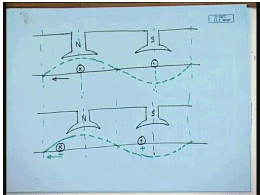Let, us say 1 conductor is below the north pole, another conductor is below the south pole. Now, the north and south pole on the stator are producing magnetic flux, and the flux density is maximum under the pole, and minimum between them, to some approximation. we can approximate, it by a the flux density to be a almost a sin wave. This is the line plot of the magnitude of the flux density over the armature surface. it is easily understandable, that in this unaligned position the net flux passing through this coil is zero. However, this armature is moving, this armature is it is moving in this direction.

So, after a while the diagram will look like, while the flux density wave form still remains maintains, it is specious pattern, that is zero. At, this points comparing these 2 diagrams, we can see that there is a change in total flux that passes through the coil, that is there is a change in flux linkage. In this case, the unaligned position total flux passing through the coil was zero. In this case, what has happened the total flux passing through the coil has become positive, because it now more flux pass through this total positive net flux passes through this coil from the armature towards the pole.

Now, So, there is a change in flux linkage, and we can define a rate of change of flux linkage with time, and according to faraday’s law, in which case there will be a voltage induced in this coil, what will be the polarity of the induced voltage the polarity of the induced voltage will be, such that it will try to oppose the flow of current in this conductor, because that is the cause, because of this current flow there is a torque generated, and that is why the body is moving, it will try to oppose the flow of current in this coil. Therefore, what will be the polarity of the induced voltage polarity of the induced voltage will be this negative this positive. So, if we look at the coil , we look at the armature coil, there will be a induced voltage in the armature, which will try to oppose the supply voltage, which is forcing the current.

(Refer Slide Time: 27:30)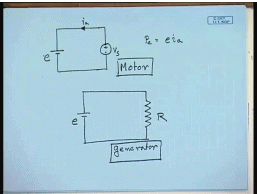Therefore, in order to send some current into the conductor some amount of electrical energy has to be spent, which is given by electrical power is given by Pe equal eia. So, this is the electrical energy accepted, and because of that there will be a torque generated in this machine, which will make this body rotate, now if we have some load torque, which may try to slow it down, and hence some mechanical work will be done.

In the process the electrical energy supplied by this source will be converted into mechanical energy, and this is called the motoring mode up operation. What will happen, if I want to make it operate as a generator in the generating mode up operation the reverse will happen in the generating mode up operation a prime mover will be used to rotate this machine. So, voltage will still be induced because there will be a rate of change of flux linkage. Now, as long as we are not connecting any resistance or any external circuit to the coil, there will be no current flowing.

Hence, there will be no repulsive force as I have indicated here repulsive or attractive force, and there will be no mechanical work done. However, as soon as I connect an external load ,which may be a resistance, then a current will start flowing the current will flow in, such a direction that it will try to slow down or stop the motor that is it will produce a torque if the current direction will be ,such that it will produce a torque, which will oppose the motion, and hence some in order to rotate the rotar against that torque some amount of mechanical energy has to be spent this mechanical energy will, then be converted into electrical energy in the form of current flowing in an external resistance, and thus mechanical energy will now be converted into electrical energy, and the electrical machine will operate as a generator.

So, this is the principle of electromagnetic electro mechanical energy conversion in a electrical machine. We see that, the same construction the same physical device can either work as a motor or as a generator depending on, which is the input if electrical energy is the input, then it will produce mechanical energy and will be called a motor. If, mechanical energy is input in the form of a prime mover, then it will produce electrical energy and it will be called a generator. So, this is the basic operating principle, but a practical d c machine will be, somewhat more complicated than the idealized picture that I have shown.

(Refer Slide Time: 31:59)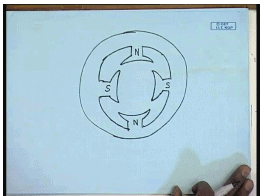Let, us look at those practical issues the stator I said carries 2 pole. However, number of pole need not be restricted to 2 you may have more number of poles. So, the pole arrangement will be north, south, north, south, this is the 1 modification in the stator that a practical machine can have the number of poles of course, will be even ,because there has to be a pair of north and south pole, but it need not be 2.

(Refer Slide Time: 33:38)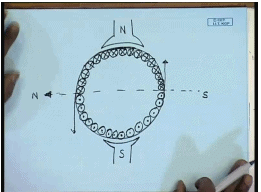Similarly, looking at the armature it will never have only 1 conductor. In fact, that will be a very poor utilization of the armature surface not only that we have seen, if I have only 1 conductor, then the force of attraction or repulsion will be maximum in this position, but as the armature rotates and comes near the aligned position the forces will reduce. So, the torque produced by this primitive machine will not be constant, but it will be highly time varying, which is not desirable.

In order to avoid that the armature is equipped with, what is called a distributed winding. So, you will have several such coils. However, the direction of current flow in half of them will be cross, and half of them will be dot naturally, because 1 is the return end of the other, but it is important that in order to, now we can understand the extend the principle of operation. For this practical case, let us say at any instant this is the polarity of current obviously, this arrangement will still produce a north pole in this direction, and a south pole in this direction, and hence there will be a force of repulsion on this body, and hence a torque will be generated.

So, the armature body with all with conductor will rotate. However, it is important thus as it rotates the overall picture of the current conduction must not change that is the top half should still be carrying all cross current the bottom half in this diagram should still be carrying dot current although the current in individual conductors may change that is a commutation arrangement has to be devised. So, that as and when 1 coil passes the commutator, it is direction of current changes this is necessary in order to maintain a uniform unidirectional torque in this machine. So, let us say how a commutator is to be arranged for this particular machine. For simplicity, we will assume.

(Refer Slide Time: 38:27)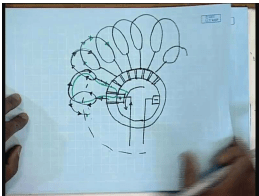Let, us say this is 1 coil all this coils in a d c machine are what we call series connected. So, this is another coil this is another coil, and this arrangement continues till the last coil closes on this first coil. So, this is called a closed winding. How, we will change the direction of current in this coils for that, we will have a commutator arrangement commutator, as I have mentioned earlier is a annular structure previously with only 1 coil we had 2 segments, but with multiple coil. Now, I should have as many segments as are as there are coils and each coil will be connected to 1 of this commutator segments, and this commutators must be insulated from each other using some insulating material.

How the commutation process takes place? Commutation process takes place when this 1 of this commutator segment is connected to the positive bus for the positive brush and another is connected to the negative brush. So, at any instant let us say current is flowing in this direction on reaching this point the current has 2 paths to flow 1 it will go this way another it will go this way. So, the current through this coil is flowing at this point in this direction through all of them and this direction.

Now, let us say this machine is moving in this direction. So, what will happen after sometime the commutator segment will move to this position this will be the new position of the commutator. please note that once the commutator comes in this position, then the coil whose 2 ends are connected to these 2 ends of the commutator are shorted. Therefore, this coil these particular coil in this case get shorted, how the current will flow then the since this is shorted there will be no current. However, the current will still flow in this direction through this coil previous as before, and through this part of the coil as before.

(Refer Slide Time: 43:06)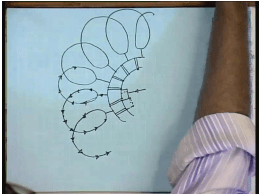Next the next instant previously, the commutator was on this segment next it has shorted this 2 as it is rotating and finally, it will arrive in this , now what will be the direction of the current current is still coming like this it is flowing like this in these coil, this was the previous position of the commutator, and in this coil it will now flow like this, comparing the direction of these 2 coils. We see that, the direction of the current that was shorted, which is said to have under 1 commutation the direction of that current in that coil has changed.

Therefore, the coil in this picture that undergoes commutation before commutation, it had a dot current, and the corresponding other side has a cross current, and the corresponding side has a dot current, then when it undergoes commutation these becomes a dot, and these becomes a cross. So, that the net all the conductors on the top side remains of cross polarity ,while all the conductor on the bottom side maintains dot polarity, and hence the overall picture and the position of the or the distribution of the magnetic flux produced by the armature winding does not change.

So, although the armature is physically rotating the flux produced by the armature winding maintains a same position. As, before and the this process the machine produces a constant unidirectional torque, 1 point is to be observed that in the process of commutation that coil that undergoes commutation is shorted for a while, and we know that ,when this coils rotate under the influence of a magnetic flux voltage is induced in them. So, if there is any voltage induced in this coil, and it is short circuited during the process of commutation there will be a very large current flowing in the coil, and it can damage the coil.

Therefore, it is not possible to short this coil when there is a induced voltage. So, only those coil in which ,the induced voltage is zero will undergo commutation this is ensured by placing the brush accordingly, the brush will connect to that conductor or that coil in which, the induced voltage is going to be zero. Now, in this diagram it will also be shown later, that in order for the induced voltage in a coil to be zero. The flux linkage of that coil should be maximum that is the coil axis should be either coinciding with the axis of the north pole of the stator or with the south pole of the stator that is the coil sides should be at what we call the interpolar region, this is the called the interpolar region, which is the middle position between 2 flux that is the coil sides should be on inter polar region, and the brush must connect to those coils, which are at the interpolar region that is ,why it is sometimes said that the brushes in a d c machine are placed in the at the interpolar position. Although, physically as we will see the brushes may not be exactly in the interpolar position, but they will connect to a coil, whose coil sides are at interpolar position physically wherever that might mean. Obviously,

(Refer Slide Time: 49:34)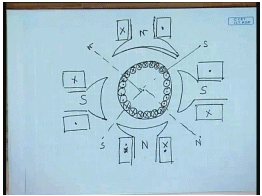If we have more than 2 poles on the armature, we have more than 2 poles on the stator. The armature should also produce equal number of poles that is, for this case we had 2 poles on the stator armature produced 2 poles. Here, armature should also produce four poles. In order, to produce poles in this fashion the armature coil current polarities should be alternating that is if these are cross from 1 pole this should be dots, then again cross then again dot. So, the armature must also be out for the same number of poles. As, the number of poles on the stator, otherwise a steady stock will not be generated.

So, the armature coils then will be will have same number of will produce same number of poles. As, the stator now these pole pairs north south 1 set of pole pair will be connected in series. So, there will be several, such pole pairs depending on the number of pole pairs on the stator, these pole pairs can be connected either in parallel or in series accordingly, there will be 2 types of armature winding called the lap winding and the wave winding, while the lap winding is series connection parallel connection of armature poles, and the wave winding is the series connection of armature poles.

We have not discussed about the possibility of connection of the stator poles these stator poles are also usually not permanent magnet, but electro magnets that gives the ability to control the strength of these magnets. Now, there is a large variety of ways in which these field coils can be connected. There is a large variety of ways in which, these field coil can be connected they can be connected in series with the armature giving rise to what is called a series machine? They can be connected to a separate source by, which we can get what is called a separately excited d c machine? they can be connected parallel to the armature by which we get a shunt d c machine in each field coil can have even 2 parts 1 part may be connected parallel to the armature, and other part can be connected in series with the armature this is called the compound field d c machine. So, depending on the connection of the pole we can have a large variety of d c machines. However, their basic operating principle remains as described. In the coming lectures, we will see a few constructional details, and the characteristics of these different types of d c machines.

Thank you.

The document Operating Principles Of DC Machines Notes | Study Electrical Machines - Electrical Engineering (EE) is a part of the Electrical Engineering (EE) Course Electrical Machines.
All you need of Electrical Engineering (EE) at this link: Electrical Engineering (EE)Use Code STAYHOME200 and get INR 200 additional OFF

## Electrical Machines

62 docs|20 tests

Track your progress, build streaks, highlight & save important lessons and more!

,

,

,

,

,

,

,

,

,

,

,

,

,

,

,

,

,

,

,

,

,

;